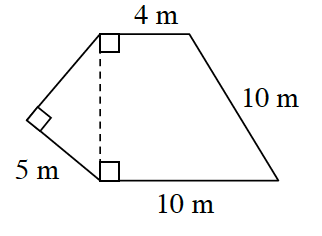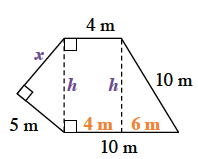Home > CCG > Chapter 7 > Lesson 7.1.3 > Problem7-39

7-39.

Find the perimeter of the shape below. Show all work. Homework Help ✎Split the shape into three different parts and label the unknown lengths you will need to find in order to ultimately find the perimeter.

Use the Pythagorean Theorem to solve for $h$.

After solving for $h$, you can use the Pythagorean Theorem again to solve for $x$.